• +91 9971497814
• info@interviewmaterial.com

# Surface Areas and Volumes Ex-13.4 Interview Questions Answers

### Related Subjects

Question 1 :

A drinking glass isin the shape of a frustum of a cone of height 14 cm. The diameters of its twocircular ends are 4 cm and 2 cm. Find the capacity of the glass.

Radius (r1) of the upper base = 4/2= 2 cm

Radius (r2) of lower the base = 2/2= 1 cm

Height = 14 cm

Now, Capacity of glass = Volume of frustum ofcone

So, Capacity of glass = (⅓)×π×h(r12+r22+r1r2)

= (⅓)×π×(14)(22+12+(2)(1))

The capacity of the glass = 102×(⅔) cm3

Question 2 :

The slant height ofa frustum of a cone is 4 cm and the perimeters (circumference) of its circularends are 18 cm and 6 cm. Find the surface area of the frustum.

Given,

Slant height (l) = 4 cm

Circumference of upper circular end of thefrustum = 18 cm

2πr1 = 18

Or, r1 = 9/π

Similarly, circumference of lower end of thefrustum = 6 cm

2πr2 = 6

Or, r2 = 6/π

Now, CSA of frustum = π(r1+r2)× l

= π(9/π+6/π) × 4

= 12×4 = 48 cm2

Question 3 :

A fez, the cap usedby the Turks, is shaped like the frustum of a cone (see Fig.). If its radius onthe open side is 10 cm, radius at the upper base is 4 cm and its slant heightis 15 cm, find the area of material used for making it.

Answer 3 :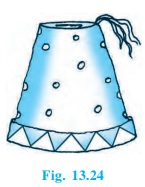Solution:

Given,

For the lower circular end, radius (r1)= 10 cm

For the upper circular end, radius (r2)= 4 cm

Slant height (l) of frustum = 15 cm

Now,

The area of material to be used for making thefez = CSA of frustum + Area of upper circular end

CSA of frustum = π(r1+r2)×l

= 210π

And, Area of upper circular end = πr22

= 16π

The area of material used = 710 × (2/7) cm2

Question 4 :

A container, openedfrom the top and made up of a metal sheet, is in the form of a frustum of acone of height 16 cm with radii of its lower and upper ends as 8 cm and 20 cm,respectively.

Answer 4 : Find the cost of the milk which can completely fill the container, at the rate of Rs. 20 per litre. Also find the cost of metal sheet used to make the container, if it costs Rs. 8 per 100 cm_2.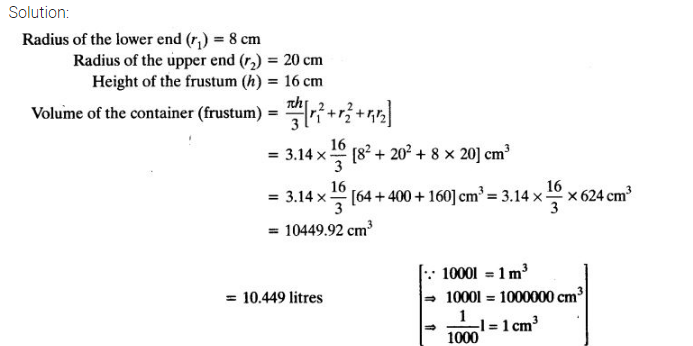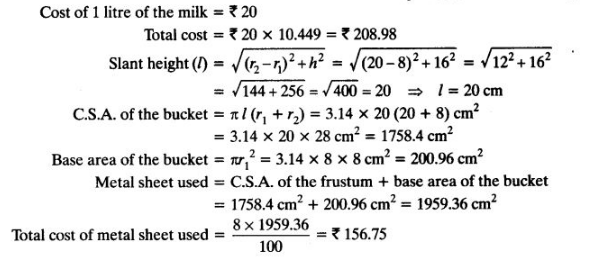Question 5 :

A metallic rightcircular cone 20 cm high and whose vertical angle is 60° is cut into two partsat the middle of its height by a plane parallel to its base.

Answer 5 :  If the frustum so obtained is drawn into a wire of diameter 1/16 cm, find the length of the wire.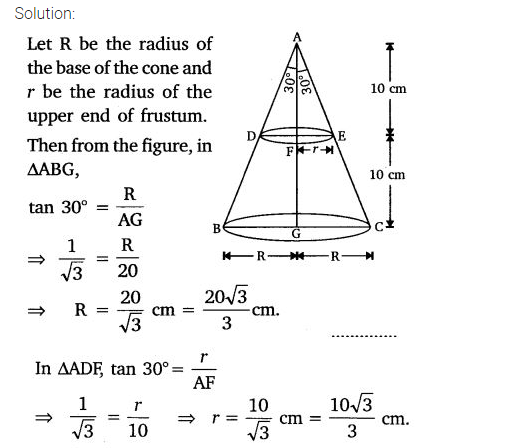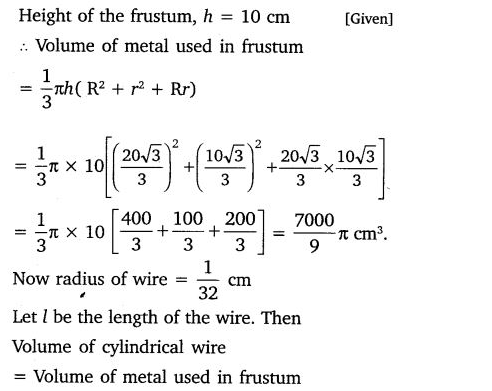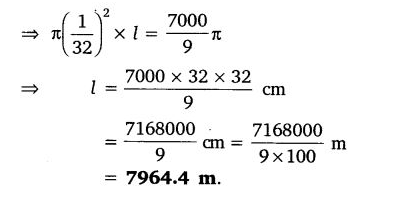Todays Deals